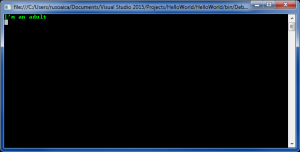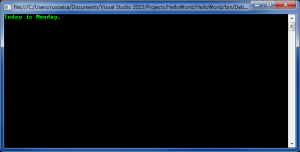Sunday, September 24, 2023 01:18

## Conditional statement If-Else

In addition to If, C# offers conditional statement If-Else. When I explained the If statement, I was saying that the program will execute the instructions only if the If condition is True. What if we wanted to execute a total different condition when and only when that condition is False?

True, we could write another If statement, checking if the condition is False and write the code there, but that would not be very elegant. Instead, we use the conditional statement If-Else:

In the above example, the program declares a variable of type int, which we initialize as 18. After that, we are checking if the age is greater than or equal to 18, and print a text if it returns True. If it returns False, we are executing a total different instruction, printing a different text.Of course, what I said about the If conditional statement applies to If Else too: when having more than one instruction after the conditional check, be it If or Else, place the instructions in a code block delimited by curly brackets:

The last form of of the If statement is If-Else If-Else. We can use this to perform two or more conditional checks, with a default action in case none of the checks returns True. The following example illustrates this case:

The output follows:From the above example, you can see that we are checking our string variable against all days of the working week, writing various texts for each scenario, and a default one in case none of the checks return True.

The concepts explained in this lesson are also shown visually as part of the following video:

EXERCISES
1. Write a program that shows the sign (+ or -) of the product of three real numbers, without calculating it. Use a sequence of if operators.

Solution

Guidelines: A multiple of non-zero numbers has a positive product, if the negative multiples are even number. If the count of the negative numbers is odd, the product is negative. If at least one of the numbers is zero, the product is also zero. Use a counter `negativeNumbersCount` to keep the number of negative numbers. Check each number whether it is negative and change the counter accordingly. If some of the numbers is 0, print “0” as result (the zero has no sign). Otherwise print “+” or “-” depending on the condition `(negativeNumbersCount % 2 == 0)`.